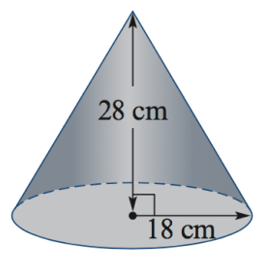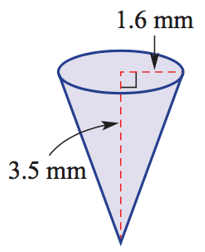PRIMARY

2 - 6

SECONDARY

7 - 10

SENIOR

11 - 12

Home

# Area of a Triangle

Theory & Practice Questions.

## Lesson

#### Volume of a Cone

A cone occupies one third of the volume of a cylinder

The volume of a cone is found by using the formula:

#### [Formula]

 V = 1/3 Ah

Where A = πr²

 V = 1/3 πr²h## Example

Calculate the volume of the cone,## Explanation

Recall the formula for calculating the volume of a cone,

V = 1/3 πr²h

Using the formula where r=18 and h=28, we substitute these numbers into the formula,

V = 1/3 π(18)²(28)

V = 3024πcm³ (exact form)

∴V ≈ 9500cm³ (approximation, correct to the nearest whole number)

## Question

Find the volume of the following cone correct to two decimal places.## Explanation

Recall the formula for the Volume of a cone.

V = 1/3 πr²h

Where r=1.6 and h=3.5

V = 1/3 π(1.6)²(3.5)

V =9.38